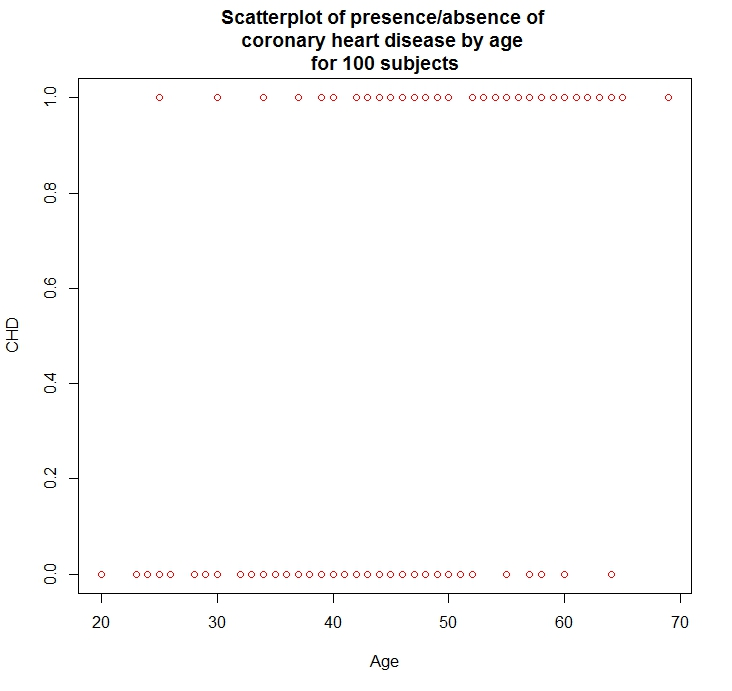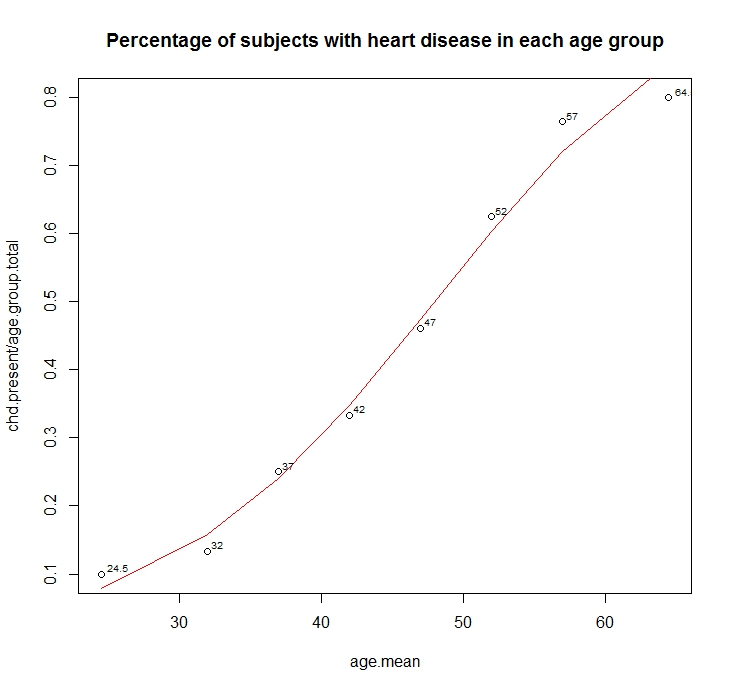# 06.23.13

## Heart-Disease Predictor Using Logistic Regression

Posted in Linear Regression at 10:48 am by Auro Tripathy

Probability is the very guide of life.
- Cicero

Given a two-column dataset, column one being age and column two being the presence/absence of heart-disease, we build a model (in R) that predicts the probability of heart-disease at an age. For a realistic model we aught to have big datasets with additional predictor variables such as blood-pressure, cholesterol, diabetes, smoking etc. However, the one-and-only predictor variable we have is age and the sample-size is 100 subjects!

Plotting the data (see below) doesn’t really provide a clear picture of the nature of the relationship between heart-disease and age. The problem is that the response variable (presence/absence of heart disease) is binary.Let’s create intervals of the independent variable (age) and compute the frequency of occurrence of the response variable (presence/absence of heart disease). You can get the table below  here.

A short and lucid tutorial in logistic regression is here (text) and here (video). The logistic curve is an S-shaped curve that takes the form,
y = [exp(b0 + b1x)] / [1 + exp(b0 + b1x)]

Clearly, the curve is non-linear, but the logit-transform makes it linear.
logit(y) = b0 + b1x

Thus, logistic regression is linear regression on the logit transform of y, where y is the probability of success at each value of x. Logistic regression fits b0 and b1, the regression coefficients.

The glm package in R is used to fit generalized regression models and can be used for logistic regression by specifying the family parameter to be binomial with the logit link like so:

```> glm.out = glm(cbind(chd.present, chd.absent) ~ age.mean,
+               family=binomial(logit), data=frequency.coronary.data)```

Plotting the fit shows us the close relationship between the fitted values and the observed values.Below is the R code that generated the plots.

```rm(list=ls())
plot(CHD ~ Age, data=coronary.data, col="red")
title(main="Scatterplot of presence/absence of \ncoronary heart disease by age \nfor 100 subjects")

library(calibrate) #needed to label observation
frequency.coronary.data[,"age.mean"] <- (frequency.coronary.data\$age.start +
frequency.coronary.data\$age.end)/2
frequency.coronary.data <- frequency.coronary.data[, c(1,2,6,3,4,5)] #reorder cols
#With "family=" set to "binomial" with a "logit" link,
# glm( ) produces a logistic regression
glm.fit = glm(cbind(chd.present, chd.absent) ~ age.mean,
family=binomial(logit), data=frequency.coronary.data)

summary(glm.fit)
plot(chd.present/age.group.total ~ age.mean, data=frequency.coronary.data)
lines(frequency.coronary.data\$age.mean, glm.fit\$fitted, type="l", col="red")

textxy(frequency.coronary.data\$age.mean,
frequency.coronary.data\$chd.present/frequency.coronary.data\$age.group.total,
frequency.coronary.data\$age.mean, cx=0.6)
title(main="Percentage of subjects with heart disease in each age group")```

Created by Pretty R at inside-R.org

References